Courses

# Test: (Previous Year Questions) - Organic Chemistry (Level 1)

## 17 Questions MCQ Test Chemistry Class 11 | Test: (Previous Year Questions) - Organic Chemistry (Level 1)

Description
This mock test of Test: (Previous Year Questions) - Organic Chemistry (Level 1) for Class 11 helps you for every Class 11 entrance exam. This contains 17 Multiple Choice Questions for Class 11 Test: (Previous Year Questions) - Organic Chemistry (Level 1) (mcq) to study with solutions a complete question bank. The solved questions answers in this Test: (Previous Year Questions) - Organic Chemistry (Level 1) quiz give you a good mix of easy questions and tough questions. Class 11 students definitely take this Test: (Previous Year Questions) - Organic Chemistry (Level 1) exercise for a better result in the exam. You can find other Test: (Previous Year Questions) - Organic Chemistry (Level 1) extra questions, long questions & short questions for Class 11 on EduRev as well by searching above.
QUESTION: 1

### In the following benzyl/alkyl system R – CH = CH2 or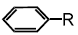(R is alkyl group) increasing order of inductive effect is –   [AIEEE-2002]

Solution:

As the branching increases so the portion tendency of CH3 group increases and therefore the order of inductive effect increases with increase in branching and so the option a is correct.

QUESTION: 2

### When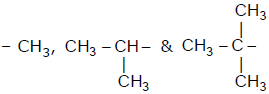groups are introduced on benzene ring then correct order of their inductive effect is -                          [AIEEE-2002]

Solution:

The correct answer is option B.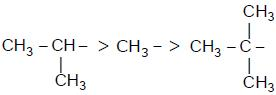After introducing it to a benzene ring: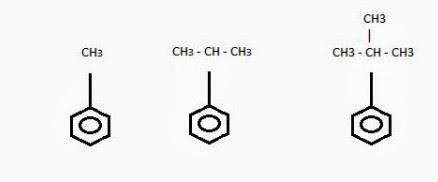The order of inductive effect is: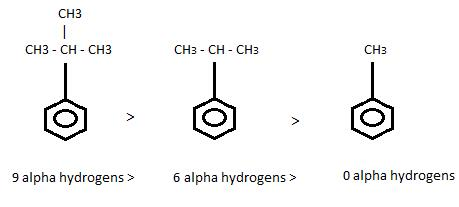+I order: 3o  > 2o  > Methyl
Reason: More number of α hydrogens.

QUESTION: 3

### The correct order of increasing basic no. of the bases NH3, CH3NH2 and (CH3)2NH is –  [AIEEE-2003]

Solution:

Except the amines containing tertiary butyl group, all lower aliphatic amines are stronger bases than ammonia because of +I (inductive) effect. The alkyl groups, which are electron releasing groups, increase the electron density around the nitrogen thereby, increasing the availability of the lone pair of electrons to proton or Lewis acids and making the amine more basic.

The observed order in the case of lower members is found to be as secondary > primary > tertiary. This anomalous behavior of tertiary amines is due to steric factors i.e. crowding of alkyl groups cover nitrogen atom from all sides and thus makes it unable for protonation. Thus the relative strength is in order (CH3)2NH > CH3NH2 > NH3.

QUESTION: 4

Rate of the reaction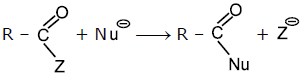is fastest when Z is -

[AIEEE-2004]

Solution:

The correct answer is option A
For the reaction to be faster the Z should be a weak nucleophile and better leaving group. Weaker bases are good leaving groups.
In the given options, Cl is the weakest conjugate base of strong acid HCl and thus will be removed by nucleophile at the fastest rate.
CH3​COO,C2​H5​O− and NH2−​ are strong conjugate bases of weak acids and therefore have weak leaving tendencies.

QUESTION: 5

Consider the acidity of the carboxylic acids :

(a) PhCOOH
(b) o – NO2C6H4COOH
(c) p – NO2C6H4COOH
(d) m – NO2C6H4COOH

Which of the following order is correct ?

[AIEEE-2004]

Solution:

For o-NO2C6H4COOH, -M effect and –I effect of –NO2 both acts (as –NO2 is closest to acid). M
Meta position, there is no –M effect of –NO2 only –I effect.
Para position –M effect of –NO2 acts but –I don’t (Para position is far from acid)
Since –NO2 is an electron-withdrawing group, it will withdraw electrons from –COOH which will release H+ to gain the electron.

QUESTION: 6

Which of the following is the strongest base -

[AIEEE-2004]

Solution:

In all 3 cases N lone pair are in resonance... and hence cannot be donated! but in 4th one N lone pair are not participating in resonance.  So it can be easily donated.  Therefore its basicity is strongest.

QUESTION: 7

The decreasing order of nucleophilicity among the nucleophiles

(a)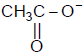(b) CH3O-

(c) CN-

(d)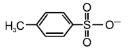is

[AIEEE-2005]

Solution:

The correct answer is option D
Conjugate base (nucleophile) is strong if acid is weak and vice-versa.
A) CH3O∣∣C−O− is a conjugate base of CH3O∣∣C−OH (I).
B) CH3O− is a conjugate base of CH3OH (II).
C) CN− is a conjugate base of HCN (III).
D) p−H3C−C6H4−SO2−O− is conjugate base of p−H3C−C6H4−SO2−OH(IV).
Order of acidic nature is IV>I>III>II.
Hence, the decreasing order of nucleophilicity is B>C>A>D.

QUESTION: 8

The reaction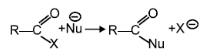is fastest when X is –

[AIEEE-2005]

Solution:

For the reaction to be faster the Z should be weak nucleophile and better leaving group. Weaker base are good leaving groups.
In the given options, Cl- is the weakest conjugate base of strong acid HCl and thus will be removed by nucleophile at the fastest rate.
CH3COO- ,C2H5O- and NH2- are strong conjugate base of weak acids and therefore have weak leaving tendency.

QUESTION: 9

Amongst the following the most basic compound is–

[AIEEE-2005]

Solution:

In aniline and in para nitro aniline lone pair of electrons are involved in delocalisation with the benzene ring and loses their basic nature. Acetanilide has electron withdrawing carbonyl group and so less basic in Benzylamine the lone pairs are readily available and hence strongly basic.

QUESTION: 10

HBr reacts with CH2 = CH – OCH3 under anhydrous conditions at room temperature to give

Solution:

H3C – CHBr – OCH3

Methyl vinyl ether is a very reactive gas. It is hydrolysed rapidly by dilute acids at room temperature to give methanol and aldehyde. However, under anhydrous conditions at room temperature, it undergoes many addition reactions at the double bond.

QUESTION: 11

CH3Br + Nu- → CH3 – Nu + Br-
The decreasing order of the rate of the above reaction with nucleophiles (Nu-) A to D is
[Nu- = (A) PhO-, (B) AcO-, (C) HO-, (D) CH3O]

[AIEEE 2006]

Solution:

The correct answer is option D
CH3​Br + Nu → CH3​ − Nu + Br
Making conjugate bases of all :
i) PHOH → H+ + PHO
Acid                    Base
(Weak)         (Strong)
ii) Ac​OH → H++ Ac​O
Acid                Base
(Weak)          (Strong)
iii) H2​O → H+ + OH
Acid                Base
(Weak)            (Strong)
iv) CH3​CH2​OH → H++ CH3​CH2​O
Acid                      Base
(Weakest)        (Strongest)
All alcohols are weaker acids than H2​O.

QUESTION: 12

The correct order of increasing acid strength of the compounds
(a) CH3CO2H

(b) MeOCH2CO2H
(c) CF3CO2H

(d)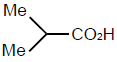is

[AIEEE 2006]

Solution:

The correct answer is option A
a.CH3​ − COOH, b.CH3​O − CH2 ​− COOH c.CF3​COOH d.(CH3​)2​CH−COOH
In the given compounds the common group is C − COOH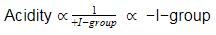Here the +I group is −CH3​
and −I groups are MeO− and F−
There are three F in the compound d, hence it is the most acidic compound.
The correct order is  d<a<b<c

QUESTION: 13

Which one of the following is the strongest base in aqueous solution ?

[AIEEE-2007]

Solution:

The increasing order of basicity of the given compounds is (CH3)2NH > CH3NH2 > (CH3)3N > C6H5NH2. Due to the +I effect of alkyl groups, the electron density on nitrogen increases and thus the availability of the lone pair of electrons to proton increases and hence the basicity of amines also increases.

So aliphatic amines are more basic than aniline. In case of tertiary anine (CH3)3N, the covering of alkyl groups over nitrogen atom from all sides makes the approach and bonding by a proton relatively difficult (steric hinderance), hence the basicity decreases. Electron withdrawing (C6H5–) groups decreases electron density on nitrogen atom and thereby decreasing basicity.

The correct option is C.

QUESTION: 14

Presence of a nitro group in a benzene ring-

[AIEEE 2007]

Solution:

From the resonating structures of nitrobenzene, it can be seen that the nitro-group withdraws electrons from the ring and hence it deactivates the benzene ring for further electrophilic substitution.

QUESTION: 15

Arrange the carbanions,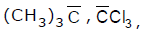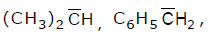in order of their decreasing stability-

[AIEEE 2009]

Solution:

1. (CH3​)3​C− is the least stable as it supply more electrons +I to the carbanion.

2. (CH3​)2​HC− is stable than (CH3​)3​C− as it has less +I effect compared to the other. But still it is less stable than the others, the reason being the same as above.

3.C6​H5​H2​C− is a resonating structure. Normally resonance effect is more compared to other effects.
But since Cl3​C has 3 Cl group −I effect, inductive effect overruns resonance effect.

QUESTION: 16

The correct order of increasing basicity of the given conjugate bases (R = CH3) is

[AIEEE 2010]

Solution:

The correct order of basicity is
RCOO- < CH≡C- < NH2- < R-
The reason behind is the electronegativity of atoms bearing negative charge. As the electronegativity of carbon is the least, it can donate its electron more freely. Similarly we can apply this on other compounds of this group. (Note that electronegativity of sp hybridised C atom is greater than sp3 hybridised N atom).

QUESTION: 17

The strongest acid amongst the following compounds is

[AIEEE 2011]

Solution:

The correct answer is option B
CH3CH2CH(Cl)COOH is the strongest acid due to the presence of one −I effect chlorine at α-carbon increases the acid strength significantly.